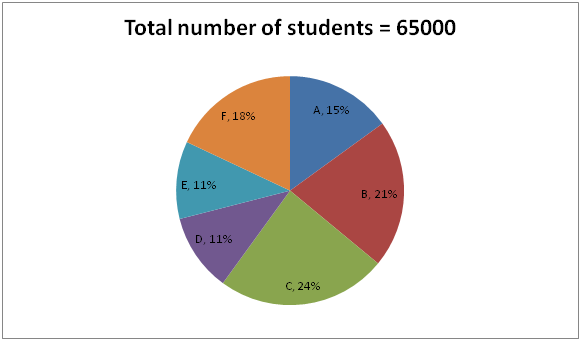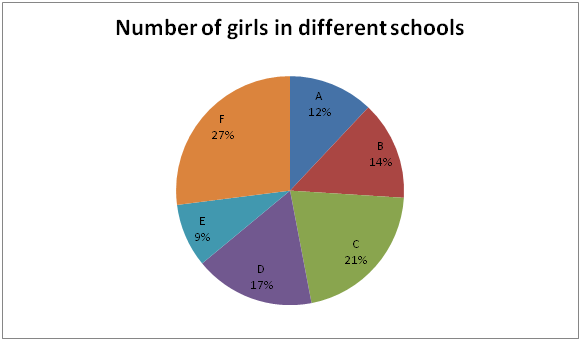# IBPS PO Mains 2018 – Quantitative Aptitude Questions Day-30

Dear Readers, Bank Exam Race for the Year 2018 is already started, To enrich your preparation here we have providing new series of Practice Questions on Quantitative Aptitude – Section. Candidates those who are preparing for IBPS PO Mains 2018 Exams can practice these questions daily and make your preparation effective.

[WpProQuiz 4312]

Directions (Q. 1 – 5) Study the following information carefully and answer the given questions:

The following pie chart1 shows the percentage distribution of total number of students in six different schools and the pie chart2 shows the percentage distribution of total number of girls among them.

The ratio of boys to girls is 7: 61) Find the difference between the total number of girls and total number of boys studying in School B?

a) 5250

b) 4800

c) 4650

d) 5500

e) None of these

2) Total number of girls studying in School A and C together is approximately what percentage of total number of boys studying in School D and E together?

a) 144 %

b) 136 %

c) 152 %

d) 118 %

e) 128%

3) What is the total number of boys studying in School B, D, E and F together?

a) 17400

b) 18250

c) 16600

d) 19550

e) None of these

4) Find the ratio between the total number of girls studying in School A, C, E together to that of boys studying in the same School?

a) 133: 181

b) 126: 199

c) 117: 173

d) 141: 219

e) None of these

5) Only 60 % and 75 % of the students in School B and C is planned to go for an excursion respectively. Find the total students didn’t go for any excursion in School B to that of Students in School C?

a) 8800

b) 7600

c) 9360

d) 10500

e) None of these

Directions (Q. 6 – 10): The following questions are accompanied by three statements I, II and III. You have to determine which statement is/are sufficient to answer the questions.

6) What is the speed of the train ‘A’?

1. Train A crosses 120 meter long train B running in opposite direction in 15 seconds.
2. Speed of train B is 72 km/hr.
3. Length of train A is thrice that of train B.

a) If statement I alone is sufficient

b) If III and either I or II are necessary

c) If I and either II or III are necessary.

d) If any two of I, II and III are necessary.

e) If all statements together are necessary

7) A person travels by car from place M to N and from N to M. How long will it take to travel from M to N by train if the speed of the train is 30% more than the speed of the car?

1. It takes 13 hours for travelling from M to N by car.
2. The distance between the two places is 650 km.
3. If he travels by bus he takes 6 hours more.

a) If I and II is sufficient

b) If III and either I or II are sufficient

c) If I and either II or III are sufficient

d) If any two of I, II and III are sufficient.

e) If all statements together are necessary

8) What is the ratio of boys and girls in the school?

1. There are 900 students in the school.
2. The number of boys in the school is 20% more than that of girls.
3. The number of girls in the school is 100 less than that of boys.

a) If either I or II is sufficient

b) If III and either I or II are sufficient

c) If I and III together or II alone is sufficient

d) If any two of I, II and III are sufficient.

e) If all statements together are necessary

9) How long will it taken by A, B and C to complete the work together?

1. A and B complete a work in 10 days
2. B and C together complete the same piece of work in 15 days.
3. A and C together complete the same piece of work in 20 days.

a) If either I or II is sufficient

b) If III and either I or II are sufficient

c) If I and either II or III are sufficient

d) If any two of I, II and III are sufficient.

e) If all statements together are necessary

10) A shopkeeper purchased an article for Rs. 300 and incurred Rs. 40 for transportation. Find the profit earned by the shopkeeper?

1. The shopkeeper marked up the price of the article by 30 %.
2. 15% discount was allowed by the shopkeeper.
3.  He marked the price of the article at Rs. 442.

a) If either I or II is sufficient

b) If III and either I or II are sufficient

c) If I and either II or III are sufficient

d) If any two of I, II and III are sufficient

e) If either I and II or II and III are sufficient

Directions (1-5):

Total number of students = 65000 and the ratio of boys to girls is 7: 6.

13’s = 65000

1’s = 5000

Total girls in all the given schools = 30000

Total boys in all the given schools = 35000

Total number of girls studying in School B

= > 30000*(14/100)

= > 4200

Total number of boys studying in School B

= > 65000 * (21/100) – 4200

= > 13650 – 4200

= > 9450

Required difference = 9450 – 4200 = 5250

Total number of girls studying in School A and C together

= > 30000*(33/100) = 9900

Total students studying in School D

= > 65000*(11/100) = 7150

Total girls studying in School D

= >30000*(17/100) =5100

Total boys studying in School D

= > 7150 – 5100 = 2050

Total students studying in School E

= > 65000*(11/100) = 7150

Total girls studying in School E

= > 30000*(9/100) = 2700

Total boys studying in School E

= > 7150 – 2700= 4450

Total number of boys studying in School D and E

= > 2050 + 4450 = 6500

Required % = (9900/6500)*100 = 152.3 % = 152 %

Total number of boys studying in School B, D, E and F together,

Total students studying in School B

= > 65000 * (21/100) = 13650

Total number of girls studying in School B

= > 30000*(14/100) = 4200

Total number of boys studying in School B

= > 13650 – 4200 = 9450

Total students studying in School D

= > 65000*(11/100) = 7150

Total girls studying in School D

= >30000*(17/100) =5100

Total boys studying in School D

= > 7150 – 5100 = 2050

Total students studying in School E

= > 65000*(11/100) = 7150

Total girls studying in School E

= > 30000*(9/100) = 2700

Total boys studying in School E

= > 7150 – 2700= 4450

Total students studying in School F

= > 65000*(18/100) = 11700

Total girls studying in School F

= > 30000*(27/100) = 8100

Total boys studying in School F

= > 11700 – 8100= 3600

Required total = 9450 + 2050 + 4450 + 3600

= > 19550

The total number of girls studying in School A, C, E

= > 30000 * (12/100) + 30000 * (21/100) + 30000 * (9/100)

= > 3600 + 6300 + 2700

= > 12600

The total number of boys studying in School A, C, E

= > [65000 * (15/100)-3600]+[65000*(24/100)-6300]+[65000*(11/100)]

= > 6150 + 9300 + 4450

= > 19900

Required ratio = 12600: 19900 = 126: 199

Only 60 % and 75 % of the students in School B and C is planned to go for an excursion

Total number of students didn’t go for any excursion in School B and C,

= > 65000*(21/100)*(40/100) + 65000*(24/100)*(25/100)

= > 5460 + 3900

= > 9360

Direction (6-10) :

By using all the statement together,

15 = (120+ 360)/[(72 + x)*(5/18)]

5x + 360 = 576

5x = 216

X = 43.2

Speed of train A = 43.2 km/hr

From statement I:

Speed of the train = 130/100 * Speed of the car

Train/Car = 13/10

Time ratio of train to car = 10: 13

Required time = 13/13*10 = 10 hours

From II:

No of girls be x and boys 120/100*x

Boys: girls = 120: 100 = 6:5

From I and III:

Total = 900

Boys + Girls = 900

Boys + Boys – 100= 900

2 Boys = 1000

=>Boys = 500

Girls = 400

Ratio = 500: 400 = 5:4

The answer can be determined by using statement I and III together or II alone is sufficient to answer the given question

From statement I, II and III,

(A + B)’s one day work = 1/10

(B + C)’s one day work = 1/8

(A + C)’s one day work = 1/6

2*(A + B + C) = 1/10 + 1/8 + 1/6

A + B + C = 13/(60*2) = 13/120

Time required by A, B and C to complete the work = 120/13 days

From statement I and II,

Marked price of the article = 340*(130/100) = Rs. 442

Selling price = 442*(85/100) = Rs 375.70

Profit= 375.70- 340= Rs 35.70

From statement II and III,

The profit can be easily determined in the same way.

Daily Practice Test Schedule | Good Luck

 Topic Daily Publishing Time Daily News Papers & Editorials 8.00 AM Current Affairs Quiz 9.00 AM Current Affairs Quiz (Hindi) 9.30 AM IBPS Clerk Prelims – Reasoning 10.00 AM IBPS Clerk Prelims – Reasoning (Hindi) 10.30 AM IBPS Clerk Prelims – Quantitative Aptitude 11.00 AM IBPS Clerk Prelims – Quantitative Aptitude (Hindi) 11.30 AM Vocabulary (Based on The Hindu) 12.00 PM IBPS Clerk Prelims – English Language 1.00 PM SSC Practice Questions (Reasoning/Quantitative aptitude) 2.00 PM IBPS PO/Clerk – GK Questions 3.00 PM SSC Practice Questions (English/General Knowledge) 4.00 PM Daily Current Affairs Updates 5.00 PM IBPS PO Mains – Reasoning 6.00 PM IBPS PO Mains – Quantitative Aptitude 7.00 PM IBPS PO Mains – English Language 8.00 PM